Project tutorial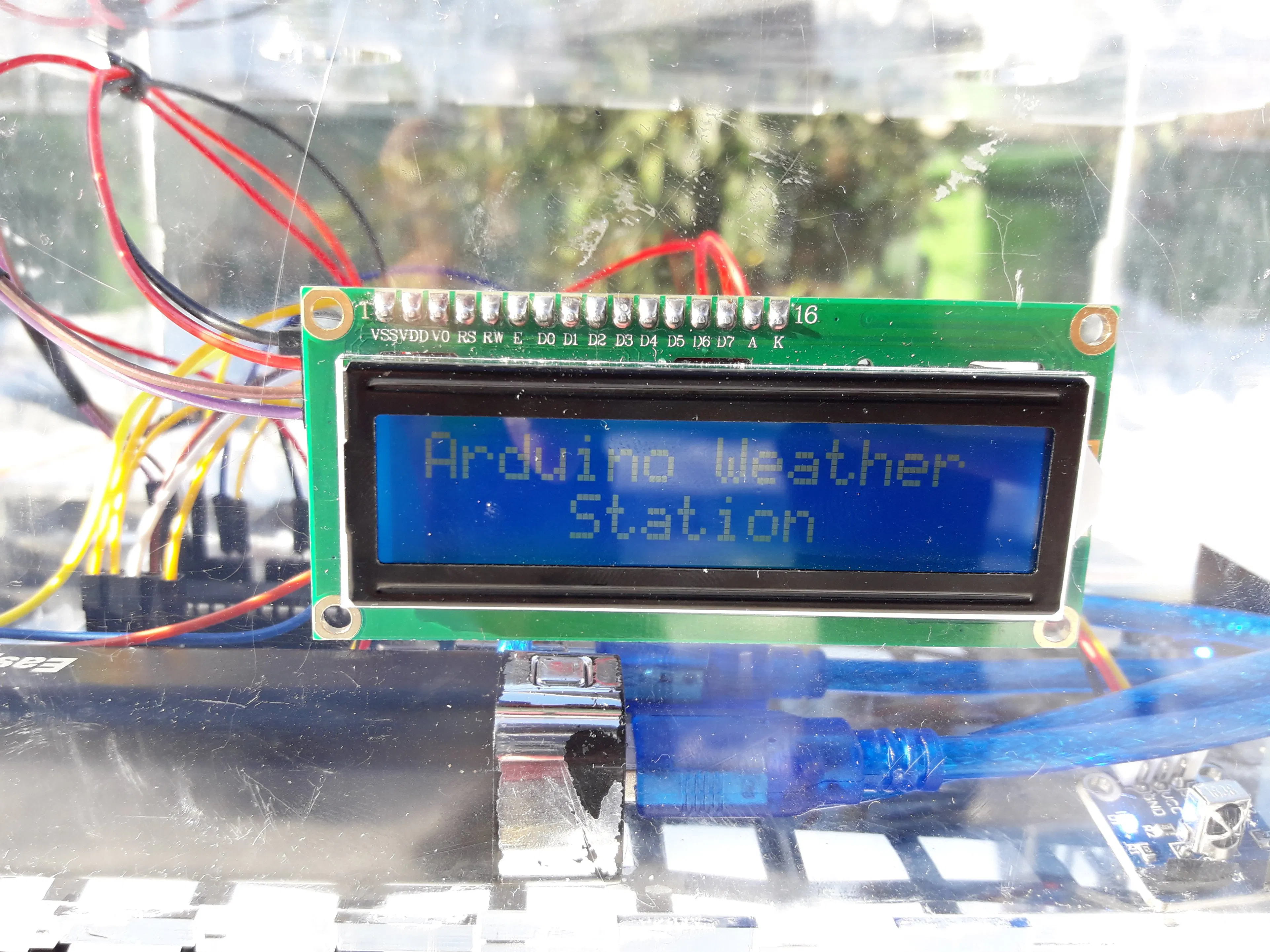AWS - Arduino Weather Station © CC BY-SA

Very useful project made at school that detects some weather data.

• 24,652 views
• 68 respects

Components and suppliesArduino UNO & Genuino UNO
×1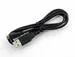USB-A to Micro-USB Cable
×1×1Jumper wires (generic)
×1
 Plexiglass 4 mm
×1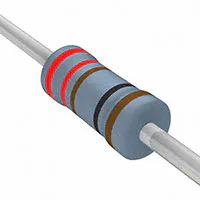Resistor 221 ohm
×1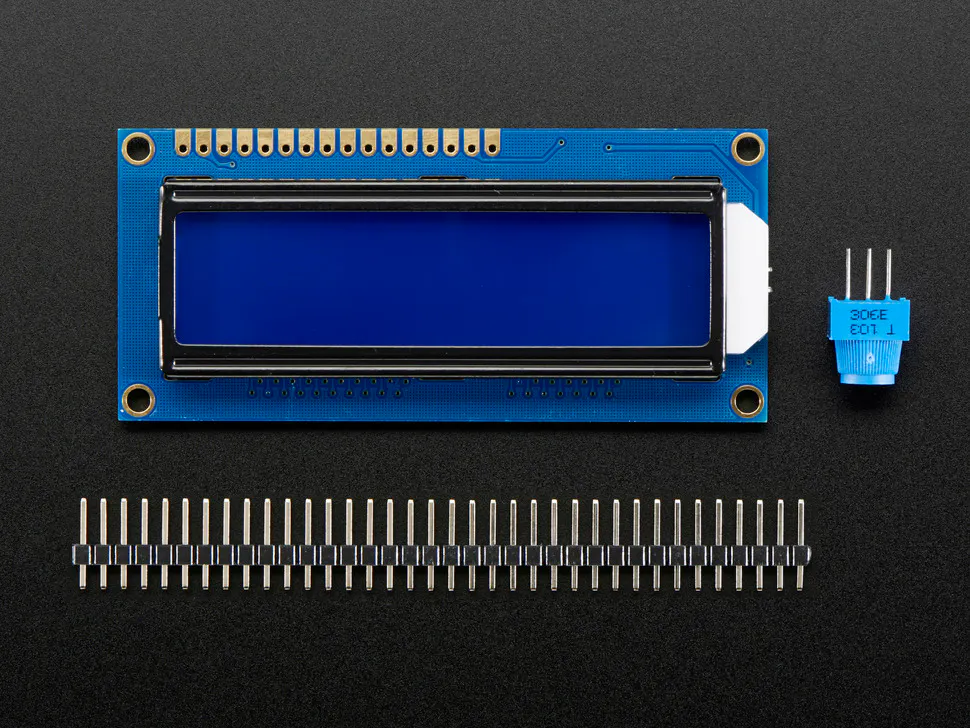Adafruit Standard LCD - 16x2 White on Blue
×1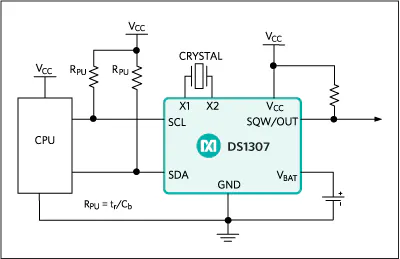Maxim Integrated DS1307 64 x 8, Serial, I²C Real-Time Clock
×1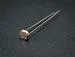Photo resistor
×1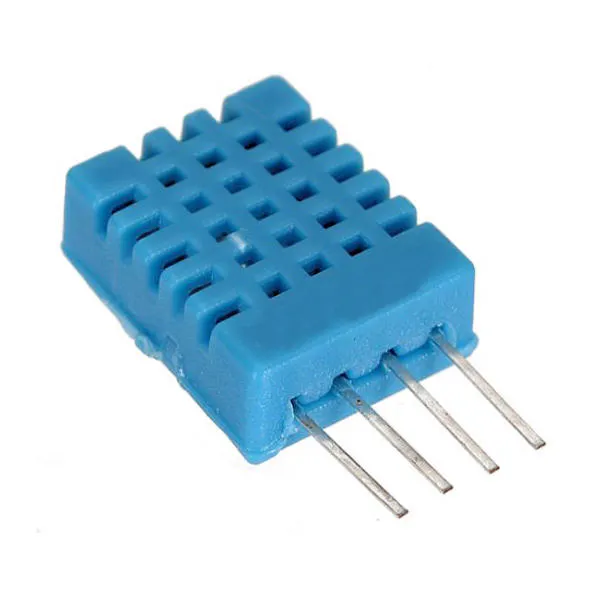DHT11 Temperature & Humidity Sensor (4 pins)
×1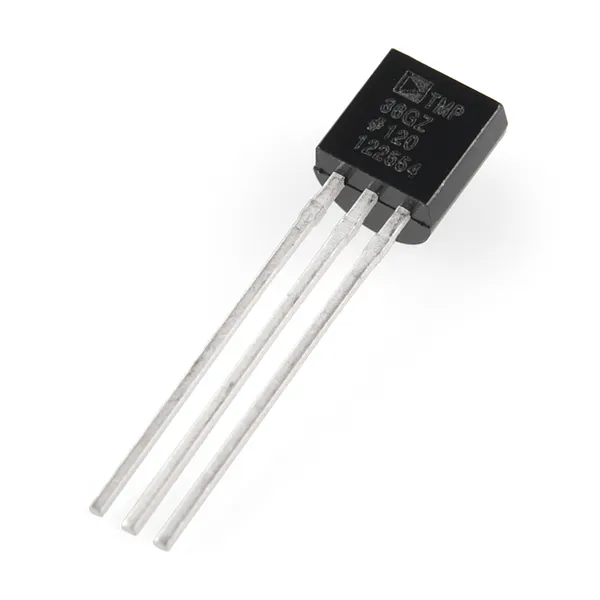Temperature Sensor
×1
×1
 Water Level Sensor
×1
 Sunfounder Starter RFID Kit
×1

Necessary tools and machines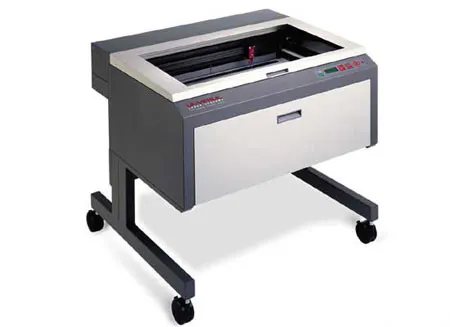Laser cutter (generic)

AWS, Arduino Weather Station, provides accurately round-the-clock data for the environment. In particular it is based on an Arduino microcontroller board, and it supports a full stuck of sensors for the analysis of the environment.

The aim

The aim of this control until is to detect a series of data through the sensors described above and to interface it with the user through an LCD screen. The little size of the project “hardware” makes it possible to closed it in a little box made with the lasercut machine.

Water and Snow Level Sensor

Water-level indicator is used to indicate the level of water in over head tank, by using this we can avoid the overflow of water, and at anytime we can know the level of water in tank.

DHT11 Humidity and Temperature Sensor

DHT11 digital temperature and humidity sensor is a calibrated digital signal output temperature and humidity combined sensor, which application-specific modules capture technology and digital temperature and humidity sensor technology to ensure that products with high reliability and excellent long-term stability. The product has excellent quality, fast response, anti-interference ability, low cost and other advantages.

Photoresistor

Photoresistors, also known as light dependent resistors (LDR), are light sensitive devices most often used to indicate the presence or absence of light, or to measure the light intensity. In the dark, their resistance is very high, but when the LDR sensor is exposed to light, the resistance drops dramatically, even down to a few ohms, depending on the light intensity.

LCD I2C 1602 Display

LCD1602 with an I2C bus is a type of serial bus. It is a high performance serial bus which has bus ruling and high or low speed device synchronization function required by multiple-host system. The blue potentiometer on the I2C LCD1602 is used toad just the backlight.

I2C uses only two bidirectional open-drain lines, Serial DataLine (SDA) and Serial Clock Line (SCL), pulled up with resistors. Typical voltages used are +5 V or +3.3 V although systems with other voltages are permitted.

DS1302 Real Time Clock Module

Itis based on the integrated DS1302 inside which there is a real-time clock /calendar and 31 bytes of static RAM. You can see the time format as hh/mm/ss, while the date format is yyyy/mm/dd.

LM35 Temperature Sensor

The LM35 Precision Temperature sensor is a sensor which detecttemperature in very accurate way. The Analog to Digital Converter (ADC)converts analog values into a digital approximation based on the formula ADCValue = sample * 1024 / reference voltage (+5v). So with a +5 volt reference, the digital approximation will = input voltage * 205.

Infrared Receiver and Remote Controller

These two components work together: the ir receiver permits the reception of data from the remote controller. The data depend on which button you want to press. The following buttons have been chosen to assign them to specific functions:

• 0 Button: you can see time and date on the LCD screen.
• 1 Button: you can see temperature data from the LM35 and humidity data from DHT11 on the LCD screen.
• 2 Button: you can see the percentage of darkness on the LCD screen.
• 3 Button: you can see the value of the fluid level (mm) on the LCD screen.
• “Cycle” Button: you can see all the screens in a time interval of 4 seconds from each other.

Step 1: Construction of the box

After considering the project size, we have proceeded with the construction of the plexiglas box. The faces sizes with their relative interlocking seats and holes for the exit of the sensor wires have been designed with Autocad software, then we have cut the faces with the lasercut machine. And finally we have assembled all with some powerful glue.

Step 2: Schematic and hardware connections

Here there is the image of schematic for the Arduino Weather Station. It has done with fritzing software. Connections are fairly simple.

Step 3: Arduinoprogramming

We have done the code on Arduino IDE, used all the libraries that we needed and programmed it so that our weather station run as we wanted.

Step 4: How itworks

AWS (Arduino Weather Station) is a weather station that picks up some data from the environment and let them possible to see by using a infrared remote controller. To interface them with the users it has an LCD display with five different screens interchangeable by the user.

These are the screens:

1. Home screen: it is the first screen that the user can see when we switch on the board. It present the project with the words “Arduino Weather Station” which have a particular fading effect. You can see this screen also after everytime you ask to AWS to seeing some data.

2. Date and Time screen: it is the first screen to which the user can access, just pressing the 0 button on the remote control. When pressed, the screen has a delay of 10 seconds and then returns to the Home screen.

3. Humidity and Temperature screen: it is the screen which give the data received by the DHT11 and LM35 sensors about humidity and temperature respectively. It is connected to the 1 button on the remote controller and when it is pressed the screen has a delay of 10 seconds and then returns to the Home screen.

4. Darkness screen: it is the screen which give the data received by the LDR about the quantity of light on the sensor. It shows the percentage of darkness on the sensor. It is connected to the 2 button on the remote controller and when pressed the screen has a delay of 10 seconds and then returns to the Home screen.

5. Fluid Level screen: this screen gives the height of a quantity of fluid into a recipe. It is connected to the 3 button on the remote controller and when it is pressed the screen has a delay of 10 seconds and then returns to the Homescreen.

The last four screens can be viewed in succession with a time interval of 4 seconds from each other, by pressing the Cycle button on the remote control. With this mode, only one lap of the information is performed.

Precautions

The first fact to be considered for the good longevity of this project is to avoid any contact of liquids or other harmful substances with the board and with the station in general.

After a precipitation it is necessary to empty the water container, check and clean the sensor.

The rechargeable battery has a duration of about two days, so it is necessary to recharge it (about 2 hours) if it is going to discharge.

Probably sometimes the infrared sensor doesn’t run as good as we want, so its function could be interrupted at low temperatures (about 0 degrees). It doesn’t represent any problem, in fact it is necessary to just open the box and press the reset button: AWS will work normally again.

If we press the reset button, the RTC DS1302 module won’t change his value about date and time, thanks to the support battery present in the module that lasts about 10 years.

If AWS power is removed, the date and time will change. To solve the problem it is necessary to re-set them in the point indicated on the list according to your preferences. Once loaded, you need to hide the settings to the program as indicated below.

Before:
Time t(2017, 12, 03, 10, 15, 00, 1);
rtc.time(t);
After:
//Time t(2017, 12, 03, 10, 15, 00, 1)//;
rtc.time(t);

Code

//include sketch libraries
#include <IRremote.h>
#include <stdio.h> //clock library
#include <string.h> //clock library
#include <DS1302.h> //clock library
#include <dht.h> //dht11 library
#include <LiquidCrystal_I2C.h> //LCD library
#include <Wire.h> //Wire for LCD library
#define lmPin A1  //LM35 attach to A1
LiquidCrystal_I2C lcd(0x27, 16, 2); // set the LCD address to 0x27 for a 16 chars and 2 line display
dht DHT; //create a variable type of dht
const int DHT11_PIN = 4; //attach dht11 to pin 4
const int waterSensor = 0; //set water sensor variable
int waterValue = 0; //variable for water sensor
int mmwaterValue = 0;
int sensorPin = A3; // select the input pin for the potentiometer
int luce = 0; //variable for the ldr
int pluce = 0; //variable for the ldr
float tem = 0; //variable for the temperature
long lmVal = 0; //variable for the LM35
//ir
const int irReceiverPin = 3;
IRrecv irrecv(irReceiverPin);  //Creates a variable of type IRrecv
decode_results results;
//define clock variable
uint8_t RST_PIN   = 5;  //RST pin attach to
uint8_t SDA_PIN   = 6;  //IO pin attach to
uint8_t SCL_PIN = 7;  //clk Pin attach to
/* Create buffers */
char buf;
char day;
/* Create a DS1302 object */
DS1302 rtc(RST_PIN, SDA_PIN, SCL_PIN);//create a variable type of DS1302
void print_time()
{
/* Get the current time and date from the chip */
Time t = rtc.time();
/* Name the day of the week */
memset(day, 0, sizeof(day));
switch (t.day)
{
case 1:
strcpy(day, "Sun");
break;
case 2:
strcpy(day, "Mon");
break;
case 3:
strcpy(day, "Tue");
break;
case 4:
strcpy(day, "Wed");
break;
case 5:
strcpy(day, "Thu");
break;
case 6:
strcpy(day, "Fri");
break;
case 7:
strcpy(day, "Sat");
break;
}
/* Format the time and date and insert into the temporary buffer */
snprintf(buf, sizeof(buf), "%s %04d-%02d-%02d %02d:%02d:%02d", day, t.yr, t.mon, t.date, t.hr, t.min, t.sec);
/* Print the formatted string to serial so we can see the time */
Serial.println(buf);
lcd.setCursor(2, 0);
lcd.print(t.yr);
lcd.print("-");
lcd.print(t.mon / 10);
lcd.print(t.mon % 10);
lcd.print("-");
lcd.print(t.date / 10);
lcd.print(t.date % 10);
lcd.print(" ");
lcd.print(day);
lcd.setCursor(4, 1);
lcd.print(t.hr);
lcd.print(":");
lcd.print(t.min / 10);
lcd.print(t.min % 10);
lcd.print(":");
lcd.print(t.sec / 10);
lcd.print(t.sec % 10);
}
void setup() {
//clock
Serial.begin(9600);
rtc.write_protect(false);
rtc.halt(false);
//ir
irrecv.enableIRIn();  //enable ir receiver module
lcd.init();  //initialize the lcd
lcd.backlight();  //open the backlight
pinMode(sensorPin, INPUT);
Time t(2017, 12, 03, 10, 15, 00, 1);//initialize the time
/* Set the time and date on the chip */
rtc.time(t);
}
void loop() {
lcd.setCursor(0, 0);
lcd.print("A");
delay(50);
lcd.setCursor(1, 0);
lcd.print("r");
delay(50);
lcd.setCursor(2, 0);
lcd.print("d");
delay(50);
lcd.setCursor(3, 0);
lcd.print("u");
delay(50);
lcd.setCursor(4, 0);
lcd.print("i");
delay(50);
lcd.setCursor(5, 0);
lcd.print("n");
delay(50);
lcd.setCursor(6, 0);
lcd.print("o");
delay(50);
lcd.setCursor(8, 0);
lcd.print("W");
delay(50);
lcd.setCursor(9, 0);
lcd.print("e");
delay(50);
lcd.setCursor(10, 0);
lcd.print("a");
delay(50);
lcd.setCursor(11, 0);
lcd.print("t");
delay(50);
lcd.setCursor(12, 0);
lcd.print("h");
delay(50);
lcd.setCursor(13, 0);
lcd.print("e");
delay(50);
lcd.setCursor(14, 0);
lcd.print("r");
delay(50);
lcd.setCursor(4, 1);
lcd.print("S");
delay(50);
lcd.setCursor(5, 1);
lcd.print("t");
delay(50);
lcd.setCursor(6, 1);
lcd.print("a");
delay(50);
lcd.setCursor(7, 1);
lcd.print("t");
delay(50);
lcd.setCursor(8, 1);
lcd.print("i");
delay(50);
lcd.setCursor(9, 1);
lcd.print("o");
delay(50);
lcd.setCursor(10, 1);
lcd.print("n");
delay(50);
if (irrecv.decode(&results)) //if the ir receiver module receiver data
{
if (results.value == 0xFF6897) //if "0" is pushed print TIME
{
lcd.clear(); //clear the LCD
print_time();
delay(10000); //delay 10000ms
lcd.clear(); //clear the LCD
delay (200); //wait for a while
irrecv.resume();    // Receive the next value
}
if (results.value == 0xFF30CF) //if "1" is pushed print TEMPERATURE and HUMIDITY
{
lcd.clear(); //clear the LCD
//READ DATA of the DHT
int chk = DHT.read11(DHT11_PIN);
// DISPLAY DATA
lcd.setCursor(0, 0);
lcd.print("Tem:");
lmVal = analogRead(lmPin);//read the value of A1
tem = (lmVal * 0.0048828125 * 100);//5/1024=0.0048828125;1000/10=100
lcd.print(tem);//print tem
lcd.print(char(223));//print the unit" ? "
lcd.print("C      ");
// Serial.println(" C");
lcd.setCursor(0, 1);
lcd.print("Hum:");
//Serial.print("Hum:");
lcd.print(DHT.humidity, 1); //print the humidity on lcd
//Serial.print(DHT.humidity,1);
lcd.print(" %      ");
//Serial.println(" %");
delay(10000); //wait for 3000 ms
lcd.clear(); //clear the LCD
delay(200); //wait for a while
irrecv.resume();    // Receive the next value
}
if (results.value == 0xFF18E7) //if "2" is pushed print the DARKNESS
{
lcd.clear(); //clear the LCD
lcd.setCursor(4, 0); //place the cursor on 4 column, 1 row
lcd.print("Darkness:");
pluce = map(luce, 0, 1023, 0, 100); //the value of the sensor is converted into values from 0 to 100
lcd.setCursor(6, 1); //place the cursor on the middle of the LCD
lcd.print(pluce); //print the percentual
lcd.print("%"); //print the symbol
delay(10000); //delay 10000 ms
lcd.clear(); //clear the LCD
delay(200); //wait for a while
irrecv.resume();    // Receive the next value
}
if (results.value == 0xFF7A85) //if "3" is pushed print the SNOW or WATER LEVEL
{
lcd.clear(); //clear the LCD
lcd.setCursor(0, 0); //place the cursor on 0 column, 1 row
lcd.print("Fluid level(mm):"); //print "Fluid level(mm):"
int waterValue = analogRead(waterSensor); // get water sensor value
lcd.setCursor(6, 1); //place cursor at 6 column,2 row
mmwaterValue = map(waterValue, 0, 1023, 0, 40);
lcd.print(mmwaterValue);  //value displayed on lcd
delay(10000); //delay 10000ms
lcd.clear(); //clear the LCD
delay(200);
irrecv.resume();    // Receive the next value
}
if (results.value == 0xFF9867) //if "PRESENTATION" is pushed print TIME, TEM and HUM, DARKNESS and S or W LEVEL one time
{
lcd.clear(); //clear the LCD
print_time();
delay(4000); //delay 10000ms
lcd.clear(); //clear the LCD
delay (200); //wait for a while
//READ DATA of the DHT
int chk = DHT.read11(DHT11_PIN);
// DISPLAY DATA
lcd.setCursor(0, 0);
lcd.print("Tem:");
lmVal = analogRead(lmPin);//read the value of A0
tem = (lmVal * 0.0048828125 * 100);//5/1024=0.0048828125;1000/10=100
lcd.print(tem);//print tem
lcd.print(char(223));//print the unit" ? "
lcd.print("C      ");
// Serial.println(" C");
lcd.setCursor(0, 1);
lcd.print("Hum:");
//Serial.print("Hum:");
lcd.print(DHT.humidity, 1); //print the humidity on lcd
//Serial.print(DHT.humidity,1);
lcd.print(" %      ");
//Serial.println(" %");
delay(4000); //wait for 3000 ms
lcd.clear(); //clear the LCD
delay(200); //wait for a while
lcd.setCursor(4, 0); //place the cursor on 4 column, 1 row
lcd.print("Darkness:");
pluce = map(luce, 0, 1023, 0, 100); //the value of the sensor is converted into values from 0 to 100
lcd.setCursor(6, 1); //place the cursor on the middle of the LCD
lcd.print(pluce); //print the percentual
lcd.print("%"); //print the symbol
delay(4000); //delay 10000 ms
lcd.clear(); //clear the LCD
delay(200); //wait for a while
lcd.setCursor(0, 0); //place the cursor on 0 column, 1 row
lcd.print("Fluid level(mm):"); //print "Fluid level(mm):"
int waterValue = analogRead(waterSensor); // get water sensor value
lcd.setCursor(6, 1); //place cursor at 6 column,2 row
mmwaterValue = map(waterValue, 0, 1023, 0, 40);
lcd.print(mmwaterValue);  //value displayed on lcd
delay(4000); //delay 10000ms
lcd.clear(); //clear the LCD
delay(200);
irrecv.resume();    // Receive the next value
}
}
}

Instead of writing "Arduino Weather Station" letter per letter, it is possible to write it with the"switch/case" mode.

CONCLUSIONS

We have done a weather station with Arduino which takes account of current date and time and picks up some environmental data to print them on an LCD screen controlled by an infrared remote controller.

Code

AWS code.inoC/C++
//include sketch libraries
#include <IRremote.h>
#include <stdio.h> //clock library
#include <string.h> //clock library
#include <DS1302.h> //clock library
#include <dht.h> //dht11 library
#include <LiquidCrystal_I2C.h> //LCD library
#include <Wire.h> //Wire for LCD library

#define lmPin A1  //LM35 attach to A1

LiquidCrystal_I2C lcd(0x27, 16, 2); // set the LCD address to 0x27 for a 16 chars and 2 line display
dht DHT; //create a variable type of dht

const int DHT11_PIN = 4; //attach dht11 to pin 4
const int waterSensor = 0; //set water sensor variable
int waterValue = 0; //variable for water sensor
int mmwaterValue = 0;
int sensorPin = A3; // select the input pin for the potentiometer
int luce = 0; //variable for the ldr
int pluce = 0; //variable for the ldr
float tem = 0; //variable for the temperature
long lmVal = 0; //variable for the LM35
//ir
const int irReceiverPin = 3;
IRrecv irrecv(irReceiverPin);  //Creates a variable of type IRrecv
decode_results results;

//define clock variable
uint8_t RST_PIN   = 5;  //RST pin attach to
uint8_t SDA_PIN   = 6;  //IO pin attach to
uint8_t SCL_PIN = 7;  //clk Pin attach to
/* Create buffers */
char buf;
char day;

/* Create a DS1302 object */
DS1302 rtc(RST_PIN, SDA_PIN, SCL_PIN);//create a variable type of DS1302

void print_time()
{
/* Get the current time and date from the chip */
Time t = rtc.time();
/* Name the day of the week */
memset(day, 0, sizeof(day));
switch (t.day)
{
case 1:
strcpy(day, "Sun");
break;
case 2:
strcpy(day, "Mon");
break;
case 3:
strcpy(day, "Tue");
break;
case 4:
strcpy(day, "Wed");
break;
case 5:
strcpy(day, "Thu");
break;
case 6:
strcpy(day, "Fri");
break;
case 7:
strcpy(day, "Sat");
break;
}
/* Format the time and date and insert into the temporary buffer */
snprintf(buf, sizeof(buf), "%s %04d-%02d-%02d %02d:%02d:%02d", day, t.yr, t.mon, t.date, t.hr, t.min, t.sec);
/* Print the formatted string to serial so we can see the time */
Serial.println(buf);
lcd.setCursor(2, 0);
lcd.print(t.yr);
lcd.print("-");
lcd.print(t.mon / 10);
lcd.print(t.mon % 10);
lcd.print("-");
lcd.print(t.date / 10);
lcd.print(t.date % 10);
lcd.print(" ");
lcd.print(day);
lcd.setCursor(4, 1);
lcd.print(t.hr);
lcd.print(":");
lcd.print(t.min / 10);
lcd.print(t.min % 10);
lcd.print(":");
lcd.print(t.sec / 10);
lcd.print(t.sec % 10);
}

void setup() {
//clock
Serial.begin(9600);
rtc.write_protect(false);
rtc.halt(false);
//ir
irrecv.enableIRIn();  //enable ir receiver module

lcd.init();  //initialize the lcd
lcd.backlight();  //open the backlight
pinMode(sensorPin, INPUT);
Time t(2017, 12, 9, 11, 20, 00, 7);//initialize the time
/* Set the time and date on the chip */
rtc.time(t);
}

void loop() {
lcd.setCursor(0, 0);
lcd.print("A");
delay(50);
lcd.setCursor(1, 0);
lcd.print("r");
delay(50);
lcd.setCursor(2, 0);
lcd.print("d");
delay(50);
lcd.setCursor(3, 0);
lcd.print("u");
delay(50);
lcd.setCursor(4, 0);
lcd.print("i");
delay(50);
lcd.setCursor(5, 0);
lcd.print("n");
delay(50);
lcd.setCursor(6, 0);
lcd.print("o");
delay(50);
lcd.setCursor(8, 0);
lcd.print("W");
delay(50);
lcd.setCursor(9, 0);
lcd.print("e");
delay(50);
lcd.setCursor(10, 0);
lcd.print("a");
delay(50);
lcd.setCursor(11, 0);
lcd.print("t");
delay(50);
lcd.setCursor(12, 0);
lcd.print("h");
delay(50);
lcd.setCursor(13, 0);
lcd.print("e");
delay(50);
lcd.setCursor(14, 0);
lcd.print("r");
delay(50);
lcd.setCursor(4, 1);
lcd.print("S");
delay(50);
lcd.setCursor(5, 1);
lcd.print("t");
delay(50);
lcd.setCursor(6, 1);
lcd.print("a");
delay(50);
lcd.setCursor(7, 1);
lcd.print("t");
delay(50);
lcd.setCursor(8, 1);
lcd.print("i");
delay(50);
lcd.setCursor(9, 1);
lcd.print("o");
delay(50);
lcd.setCursor(10, 1);
lcd.print("n");
delay(50);

if (irrecv.decode(&results)) //if the ir receiver module receiver data
{
if (results.value == 0xFF6897) //if "0" is pushed print TIME
{
lcd.clear(); //clear the LCD
print_time();
delay(10000); //delay 10000ms
lcd.clear(); //clear the LCD
delay (200); //wait for a while
irrecv.resume();    // Receive the next value
}
if (results.value == 0xFF30CF) //if "1" is pushed print TEMPERATURE and HUMIDITY
{
lcd.clear(); //clear the LCD
//READ DATA of the DHT
int chk = DHT.read11(DHT11_PIN);
// DISPLAY DATA
lcd.setCursor(0, 0);
lcd.print("Tem:");
lmVal = analogRead(lmPin);//read the value of A1
tem = (lmVal * 0.0048828125 * 100);//5/1024=0.0048828125;1000/10=100
lcd.print(tem);//print tem
lcd.print(char(223));//print the unit"  "
lcd.print("C      ");
// Serial.println(" C");
lcd.setCursor(0, 1);
lcd.print("Hum:");
//Serial.print("Hum:");
lcd.print(DHT.humidity, 1); //print the humidity on lcd
//Serial.print(DHT.humidity,1);
lcd.print(" %      ");
//Serial.println(" %");
delay(10000); //wait for 3000 ms
lcd.clear(); //clear the LCD
delay(200); //wait for a while
irrecv.resume();    // Receive the next value
}
if (results.value == 0xFF18E7) //if "2" is pushed print the DARKNESS
{
lcd.clear(); //clear the LCD
lcd.setCursor(4, 0); //place the cursor on 4 column, 1 row
lcd.print("Darkness:");
pluce = map(luce, 0, 1023, 0, 100); //the value of the sensor is converted into values from 0 to 100
lcd.setCursor(6, 1); //place the cursor on the middle of the LCD
lcd.print(pluce); //print the percentual
lcd.print("%"); //print the symbol
delay(10000); //delay 10000 ms
lcd.clear(); //clear the LCD
delay(200); //wait for a while
irrecv.resume();    // Receive the next value
}
if (results.value == 0xFF7A85) //if "3" is pushed print the SNOW or WATER LEVEL
{
lcd.clear(); //clear the LCD
lcd.setCursor(0, 0); //place the cursor on 0 column, 1 row
lcd.print("Fluid level(mm):"); //print "Fluid level(mm):"
int waterValue = analogRead(waterSensor); // get water sensor value
lcd.setCursor(6, 1); //place cursor at 6 column,2 row
mmwaterValue = map(waterValue, 0, 1023, 0, 40);
lcd.print(mmwaterValue);  //value displayed on lcd
delay(10000); //delay 10000ms
lcd.clear(); //clear the LCD
delay(200);
irrecv.resume();    // Receive the next value
}

if (results.value == 0xFF9867) //if "PRESENTATION" is pushed print TIME, TEM and HUM, DARKNESS and S or W LEVEL one time
{
lcd.clear(); //clear the LCD
print_time();
delay(4000); //delay 10000ms
lcd.clear(); //clear the LCD
delay (200); //wait for a while

//READ DATA of the DHT
int chk = DHT.read11(DHT11_PIN);
// DISPLAY DATA
lcd.setCursor(0, 0);
lcd.print("Tem:");
lmVal = analogRead(lmPin);//read the value of A0
tem = (lmVal * 0.0048828125 * 100);//5/1024=0.0048828125;1000/10=100
lcd.print(tem);//print tem
lcd.print(char(223));//print the unit"  "
lcd.print("C      ");
// Serial.println(" C");
lcd.setCursor(0, 1);
lcd.print("Hum:");
//Serial.print("Hum:");
lcd.print(DHT.humidity, 1); //print the humidity on lcd
//Serial.print(DHT.humidity,1);
lcd.print(" %      ");
//Serial.println(" %");
delay(4000); //wait for 3000 ms
lcd.clear(); //clear the LCD
delay(200); //wait for a while

lcd.setCursor(4, 0); //place the cursor on 4 column, 1 row
lcd.print("Darkness:");
pluce = map(luce, 0, 1023, 0, 100); //the value of the sensor is converted into values from 0 to 100
lcd.setCursor(6, 1); //place the cursor on the middle of the LCD
lcd.print(pluce); //print the percentual
lcd.print("%"); //print the symbol
delay(4000); //delay 10000 ms
lcd.clear(); //clear the LCD
delay(200); //wait for a while

lcd.setCursor(0, 0); //place the cursor on 0 column, 1 row
lcd.print("Fluid level(mm):"); //print "Fluid level(mm):"
int waterValue = analogRead(waterSensor); // get water sensor value
lcd.setCursor(6, 1); //place cursor at 6 column,2 row
mmwaterValue = map(waterValue, 0, 1023, 0, 40);
lcd.print(mmwaterValue);  //value displayed on lcd
delay(4000); //delay 10000ms
lcd.clear(); //clear the LCD
delay(200);
irrecv.resume();    // Receive the next value
}
}
}

Custom parts and enclosures

box_a5et5f4U9F.dwg

Schematics

aws_DMDwb4iUZp.fzz

Wireless weather station (Arduino + ESP8266 + Thingspeak)

Project tutorial by Alin-Constantin Paun

• 8,865 views
• 11 respects

Arduino Weather Station v1.0 (BMP280)

Project tutorial by Gabriele Scordamaglia

• 7,311 views
• 43 respects

Arduino Weather Station

Project tutorial by woutvdr

• 14,237 views
• 44 respects

Personal Weather Station (Arduino+ ESP8266 + Thingspeak)

Project tutorial by Jayraj Desai

• 51,517 views
• 107 respects

Uno Weather Station

Project in progress by wrightmac

• 8,247 views